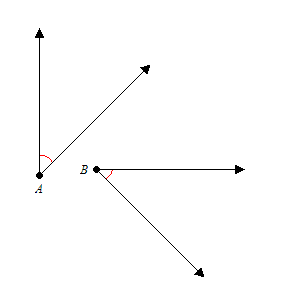# Congruent Angles

Congruent angles are angles with exactly the same measure.

Example:

In the figure shown, $\angle A$ is congruent to $\angle B$ ; they both measure $45°$ .Congruence of angles in shown in figures by marking the angles with the same number of small arcs near the vertex (here we have marked them with one red arc).

In geometric notation, if $\angle A$ is congruent to $\angle B$ , we write

$\angle A\cong \angle B$ .